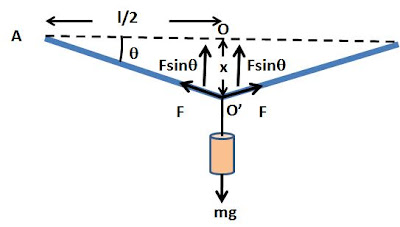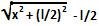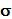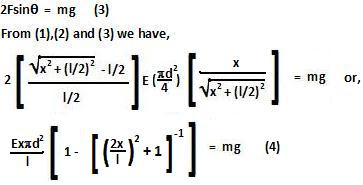## Saturday, April 4, 2009

### Irodov Problem 1.294The stress F in the wire opposes the weight of the hanging mass mg and keeps it suspended as shown in the figure. The stress in the wire comes from the fact that as the length of the wire has increased from its original state due to the mass being suspended at a distance x below the horizontal as depicted in the figure (AO <>).

theorem, thus the change in length is. The stressinduced in the string is given by,The force in the string due to the stress is the stress times the area of the thickness of the string and is given by,The total vertical component of the forces due to the both the halves of the string are responsible for holding the weight in place and so we have,(4) is not easy to solve and so we can get an approximate solution by using Maclaurin's series as follows,